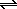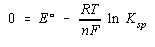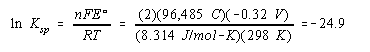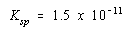Practice Problem 12

Use the following standard-state cell potentials to calculate the solubility product at 25oC for Mg(OH)2.

 Mg(OH)2 + 2 e-Mg + 2 OH- Eored = -2.69 V Mg2+ + 2 e-Mg Eored = -2.375 V

Solution

This time we have to reverse the second half-reaction to obtain an overall equation that corresponds to the appropriate equilibrium expression.

 Mg(OH)2 + 2 e-Mg + 2 OH- Eored = -2.69 V + MgMg2+ + 2 e- Eoox = 2.375 V ¯¯¯¯¯¯¯¯¯¯¯¯¯¯¯¯¯¯¯¯¯¯¯ ¯¯¯¯¯¯¯¯¯¯¯¯¯ Mg(OH)2Mg2+ + 2 OH- Eo = -0.32 V

Once again, we start with the Nernst equation and assume that the reaction is at equilibrium.We then rearrange this equation, substitute what we know about the reaction into this equation, and calculate the value of Ksp for this compound.